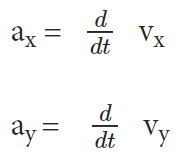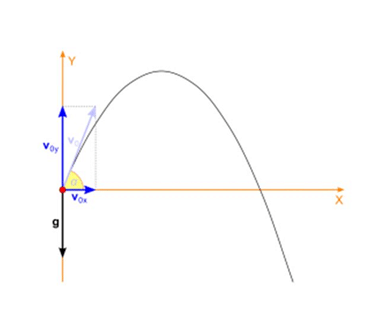NEET  >  Introduction to Motion in a Plane

# Introduction to Motion in a Plane - Physics Class 11 - NEET

 Table of contentsIntroduction to Plane MotionMotion in a PlaneProjectile Motion: Plane MotionSolved Examples for YouThe physical quantities like work, temperature and distance can be represented in day to day life wholly by their magnitude alone. However, the relation of these physical quantities can be explained by the laws of arithmetic. In order to represent physical quantities like acceleration, displacement, and force, the direction is equally essential along with the magnitude. Let us now study Plane Motion.

## Introduction to Plane Motion

Velocity refers to a physical vector quantity which is described by both magnitude and direction. The magnitude or scalar absolute value of velocity is referred to as speed. As stated by the Pythagorean Theorem, the magnitude of the velocity vector is given by –
| v | = v =  √ ( vx ²+ vy ² )
Acceleration is defined by the rate of change of velocity of an object with respect to time. Numerically or in terms of components, it can be presented as –## Motion in a Plane

Motion in a plane is also referred to as a motion in two dimensions. For example, circular motion, projectile motion, etc. For the analysis of such type of motion, the reference point will be made of an origin and the two coordinate axes X and Y.
Motion in a plane refers to the point where we consider motion in two dimensions as only two dimensions makes a plane. Here, considering the above, we take two axes into consideration – generally X-axis or Y – axes. In an attempt to derive the equation of the motion in a plane, we must know about motion in one direction.

### Equations of Plane Motion

The equations of motion in a straight line are:
v = u+at
s = ut+1/2 at²
v2 = u² + 2as
Where,

• v = final velocity of the particle
• u = initial velocity of the particle
• s = displacement of the particle
• a = acceleration of the particle
• t = the time interval in which the particle is in consideration

In a plane, we have to apply the same equations separately in both the directions: Y axis and Y-axis. This would give us the equations for motion in a plane.
vy   = u+ ayt
sy = ut +1/2 ay t²
= u²y+2as
Where,

• vy = final velocity of the particle in the y-direction
• uy = initial velocity of the particle in the y-direction
• sy = displacement of the particle in the y-direction
• ay = acceleration of the particle in the y-direction

Similarly, for the X-axis:
vx = u+ at
sx=  ux t+1/2 ax
v²x = u²x+2 axs

Where,

• v= final velocity of the particle in the x-direction
• ux = initial velocity of the particle in the x-direction
• sx= displacement of the particle in the x-direction
• a= acceleration of the particle in x-direction

## Projectile Motion: Plane Motion

One of the most common examples of motion in a plane is Projectile motion. In a projectile motion, the only acceleration acting is in the vertical direction which is acceleration due to gravity (g). Therefore, equations of motion can be applied separately in the X-axis and Y-axis to find the unknown parameters.Projectile Motion

The above diagram represents the motion of an object under the influence of gravity. It is an example of projectile motion (an special case of motion in a plane).

### Examples of  Two-Dimensional Plane Motion

• Throwing a ball or a cannonball
• The motion of a billiard ball on the billiard table.
• A motion of a shell fired from a gun.
• A motion of a boat in a river.
• The motion of the earth around the sun.

## Solved Examples for You

Q.1: The state with reasons, whether the following algebraic operations with scalar and vector physical quantities are meaningful:

• adding a scalar to a vector of the same dimensions,
• multiplying any vector by any scalar,
• multiplying any two scalars,
• adding a component of a vector to the same vector.

Ans:

• Meaningful; The addition of two scalar quantities is meaningful only if they both represent the same physical quantity.
• Not Meaningful; The addition of a vector quantity with a scalar quantity is not meaningful.
• Meaningful; A scalar can be multiplied with a vector. For example, force is multiplied with time to give impulse.
• It’s meaningful; A scalar, irrespective of the physical quantity it represents, can be multiplied by another scalar having the same or different dimensions.
• Meaningful; The addition of two vector quantities is meaningful only if they both represent the same physical quantity.
• Meaningful; A component of a vector can be added to the same vector as they both have the same dimensions.

Q.2: Read each statement below carefully and state with reasons, if it is true or false:
(a) The magnitude of a vector is always a scalar,
(b) each component of a vector is always a scalar,
Ans:

(a) True. The magnitude of a vector is a number. Hence, it is a scalar.
(b) False. Each component of a vector is also a vector.

The document Introduction to Motion in a Plane | Physics Class 11 - NEET is a part of the NEET Course Physics Class 11.
All you need of NEET at this link: NEET

## FAQs on Introduction to Motion in a Plane - Physics Class 11 - NEET

 1. What is plane motion?Ans. Plane motion refers to the motion of an object in a two-dimensional space, where the object can move in any direction within that plane.
 2. What is projectile motion?Ans. Projectile motion is a specific type of plane motion where an object is launched into the air and moves along a curved path under the influence of gravity. It is characterized by both horizontal and vertical motion.
 3. How is projectile motion different from other types of plane motion?Ans. Projectile motion differs from other types of plane motion because it involves a combination of both horizontal and vertical motion. In other types of plane motion, the object may only move in one direction within the plane.
 4. What factors affect the trajectory of a projectile?Ans. The trajectory of a projectile is affected by several factors, including the initial velocity, angle of projection, and the force of gravity. Air resistance can also have a small effect on the trajectory.
 5. Can a projectile motion be described using equations?Ans. Yes, projectile motion can be described using equations derived from the principles of motion. These equations can help determine the range, time of flight, maximum height, and other properties of the projectile's motion.

## Physics Class 11

130 videos|483 docs|210 tests

## Physics Class 11

130 videos|483 docs|210 tests

### How to Prepare for NEET

Read our guide to prepare for NEET which is created by Toppers & the best Teachers
Signup to see your scores go up within 7 days! Learn & Practice with 1000+ FREE Notes, Videos & Tests.
10M+ students study on EduRev
Track your progress, build streaks, highlight & save important lessons and more!(Scan QR code)
Related Searches

,

,

,

,

,

,

,

,

,

,

,

,

,

,

,

,

,

,

,

,

,

;